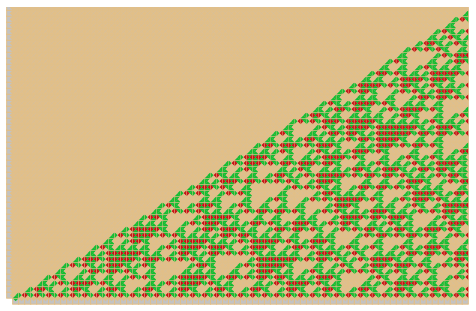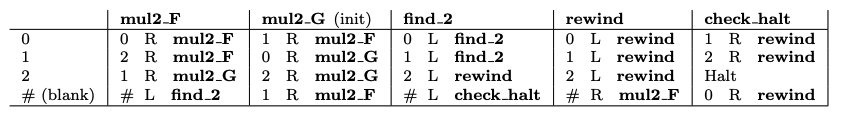We have a new paper on the hardness of knowing busy beaver values BB(15) and BB(5,4).

## Busy beaver Turing machines

When thinking abstractly about algorithms, we can ask the following question: what is the maximum number of steps that an algorithm (taking no inputs) can perform before halting (given that it is halting) when we constrain its size (e.g. the number of bytes of the source code if we wrote the algorithm in C)? Although the question is theoretical, we can argue that it is in fact very relatable to programmers as they acquire, with their everyday practice, a certain virtuosity in the art of writing small programs that achieve a lot (this even gives rise to competitions).

In order to formally study this question, we cannot escape from having to fix the precise model of computation that we work with (which can be thought as fixing the programming language that we use) and our notion of program size. The historical model used to study the question are 1-tape (bi-infinite) 2-symbols deterministic Turing machines and program size is defined by the number of states times symbols.

In this context, Tibor Radó  concretely instantiated the question by introducing the busy beaver value BB$$(n)$$ which corresponds to the maximum number of steps performed by a halting Turing machine with $$n$$ states starting from all-blank input tape ( using the precise Turing machine model described above and where one of the 2 tape symbols is called "blank"). Formally:

$$\text{BB}(n) = \max_{M \in \text{TM}(n), \; s(M) < \infty} s(M)$$

With TM$$(n)$$ the set (finite) of $$n$$-state Turing machines and $$s(M)$$ the number of steps taken by machine $$M$$ (starting from blank input) before halting or $$+\infty$$ if it doesn't halt. Brady  generalised the notion to BB$$(n,k)$$ where $$k$$ is the number of tape symbols (i.e. BB$$(n)$$ = BB$$(n,2)$$). Find more details in Section 2 of our paper or in this nice introduction (and more) by Scott Aaronson.

To date, only four non-trivial busy beaver values are known: $$\text{BB}(2) = 6$$, $$\text{BB}(3) = 21$$, $$\text{BB}(4) = 107$$, and $$\text{BB}(2,3) = \text{38}$$. It is conjectured that $$\text{BB}(5) = \text{47,176,870}$$.

One possible approach to study $$\text{BB}(n)$$ is to look for busy beaver champions i.e. machines that run for more steps than any other known machine in their (states,symbols) category. Busy beaver champions give lower bounds on busy beaver values. For instance, the current 5-state 2-symbol champion halts after 47,176,870 steps, which gives $$\text{BB}(5) \geq \text{47,176,870}$$. Pascal Michel's website and survey or Heiner Marxen website are great resources on that topic.

The route we take to study $$\text{BB}(n)$$ is different. Given that so few values of BB are currently known, we ask (as others before us): How hard is it to know $$\text{BB}(n,k)$$ for given $$n,k$$ pairs?

## Knowability of busy beaver values

As it turns out, the busy beaver function $$n \mapsto \text{BB}(n)$$ is not computable. In other words, there is no algorithm that can output $$\text{BB}(n)$$ when given $$n$$ as input: no algorithm that can give us the knowledge of $$\text{BB}(n)$$ in general. The reason is because having a procedure to compute $$n \mapsto \text{BB}(n)$$ (or any $$n \mapsto \text{BB}(n,k)$$ with $$k$$ fixed) would give rise to a procedure to decide the halting problem (on blank input) because if a machine $$M$$ with $$n$$ states and $$k$$ symbols runs for more than $$\text{BB}(n,k)$$ steps then we know for a fact that it will never halt.

This fact is a first major obstacle in trying to acquire the knowledge of $$n \mapsto \text{BB}(n)$$ as we cannot rely on a general algorithmic procedure to do so. We are only left with the hope of being able to study individual instances of $$\text{BB}(n)$$ (or $$\text{BB}(n,k)$$) and possibly find their value.

However, this dim hope is even further reduced by making the following observation: it is not that hard to design machines that look for counterexamples of notoriously hard mathematical problems. For instance, take one of the oldest open problem in mathematics, Goldbach's conjecture: every even integer greater than 2 is the sum of two primes. It is conceptually completely feasible to design a program that halts if and only if the conjecture is false: iterate over all even integer greater than 2 and test the conjecture for each then halt if a counterexample is found.

This was done in practice and a 4,888-state Turing machine that halts if and only Goldbach's conjecture is false was constructed. (Since improved to 27-states, although, we believe, yet to be proven correct.) This construction gives an algorithm to solve Goldbach's conjecture! Run the 4,888-state machine for $$\text{BB}(4,888) + 1$$ steps (this must be an unbelievably big number of steps), and if it hasn't stopped by then it means that Goldbach's conjecture is true. In that sense knowing $$\text{BB}(4,888)$$ is harder than solving Goldbach's conjecture. Hence it suggests that having a proof of the value of $$\text{BB}(4,888)$$ is harder than having a proof of Goldbach's conjecture, which is already considered as extremely hard given current mathematical knowledge.

Even worse, value $$\text{BB}(7,910)$$ has been shown to be independent of ZFC meaning that standard mathematical tools are not powerful enough to decide the value. This was later improved to $$\text{BB}(748)$$. Scott Aaronson conjectures that as low as $$\text{BB}(20)$$ is independent of ZFC and that $$\text{BB}(10)$$ is independent of Peano Arithmetic.

This all sounds like bad news for us to ever know even small values of $$\text{BB}$$. Although it does not tell us why knowing a value as small as $$\text{BB}(5)$$ should be hard, it does tell is that hardness is not all that far away as we traverse up the BB values.

In this context, there is a legitimate question to ask: where is the frontier between knowable and unknowable values of $$\text{BB}$$ situated? Our contribution to answering this question is to relate $$\text{BB}(15)$$ (and $$\text{BB}(5,4)$$) to an open problem in mathematics formulated by Paul Erdős : For all $$n>8$$ there is at least one digit 2 in the base 3 representation of $$2^n$$. This open problem was considered by Terrence Tao as a possible toy model for the weak Collatz conjecture.

Our work gives, to date, the smallest value of BB that relates to a natural open problem in mathematics.

## BB(15) and BB(5,4) are harder than Erdős' conjecture on powers of twoFigure 1. The first 75 powers of two assembled in base 3 by the size-6 Wang tile set introduced by Cook, Stérin and Woods  (see blog post). Reading left-to-right, each column of glues (colours) corresponds to a power of two: beige glues represent ternary digit 0, green glues 1 and red glues 2. For instance, the rightmost column encodes (from top to bottom) 1102100210202020112020120000201120010210212220223 = 275. The complexity of the pattern illustrates the complexity of answering Erdős' conjecture which amounts to asking if each glue-column (except for the few first) has at least one red glue.

Erdős' conjecture on powers of two is: For all $$n>8$$ there is at least one digit 2 in the base 3 representation of $$2^n$$. Although the conjecture is simple to express, Figure 1 helps to visualise the complexity behind it: patterns created by base 3 digits of powers of 2 seem chaotic to the point that even knowing if there is one red glue (digit 2) per column (power of two) is hard.

In our paper we explicitly construct two machines, one with 15 states and 2 symbols and the other with 5 states and 4 symbols that halt if and only if they have found a counterexample to Erdős' conjecture on powers of two.Figure 2. 5-state 4-symbol Turing machine M5,4 that halts if and only if Erdős’ conjecture on powers of 2 is false. The initial state of the machine is mul2_G, denoted ‘(init)’. The blank symbol is #.

## Proving correctness of our busy beaver candidates

It can be a challenge to prove correctness for programs that exploit clever and nasty code re-use tricks to be as short as we can make them. But, without a proof there is no result! Here's how we approached that problem.

We first found the 5-state 4-symbol machine, $$M_{5,4}$$. The machine is given in Figure 2 (Figure 3 in the paper) and works by simulating the 2-state Finite-state Transducer that computes multiplication in base 3 (states mul2_F, mul2_G in the machine) and then checks whether at least one digit 2 is present in the expression (state find_2). A counter is used by the machine to keep track of the three special cases of the conjecture (justifying the bound $$n > 8$$ in the statement): 1, 4 and 256 with respective base-3 representations 1, 11 and, 100111. Our Theorem 2 shows that the behavior of $$M_{5,4}$$ is correct: it halts if and only if Erdős’ conjecture is false. The proof of correctness for $$M_{5,4}$$ makes heavy use of the FST.

After constructing $$M_{5,4}$$ we translated it to a machine using only 2 symbols, $$a$$ and $$b$$ (blank symbol is $$b$$), by encoding symbols #,0,1,2 in the following way: E(#)$$= bb$$, $$E(0) = ba$$, $$E(1) = ab$$ and $$E(2) = aa$$. We further optimized the program a little to get the machine $$M_{15,2}$$ given in Figure 4 of the paper. $$M_{15,2}$$ uses 15 states and 2 symbols, and we give a (straightforward) proof that it simulates the behavior of $$M_{5,4}$$. The simulation is tight (linear time): for each step of $$M{5,4}$$ there is a short sequence of steps of $$M_{15,2}$$. This simulation,

together with Theorem 2, implies that $$M_{15,2}$$ halts if and only if Erdős’ conjecture is false.

## Conclusion

Given the conceptual simplicity of the conjecture, it is perhaps not surprising that we managed to find these small counterexample-hunting machines. In turn, this means that knowing busy beaver values as small as $$\text{BB}(15)$$ is a hard, at least as hard as solving Erdős' conjecture on powers of two as one pathway to solve the conjecture is to run $$M_{15,2}$$ for $$\text{BB}(15)+1$$ steps, if it has not halted by that time then it never halts and the conjecture is true, otherwise it is false since a counterexample was found. Although not tractable in practice, the argument gives a finite bound on Erdős' conjecture possible counterexamples.

Our result leaves open the question of where exactly is situated the frontier between knowable and unknowable busy beaver values. However, it suggests that this frontier might even be below $$\text{BB}(15)$$.

## Simulator

We made an online simulator that can be used to run the machines of the paper and verify the constructions, it is available at this address: https://dna.hamilton.ie/tsterin/bbsim/. You can also use it to simulate your own machines, code and documentation are available here.